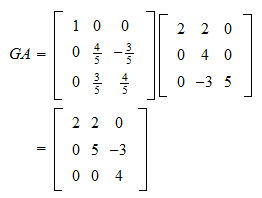StatLect

# Givens rotation matrix

The Givens rotation matrix (or plane rotation matrix) is an orthogonal matrix that is often used to transform a real matrix into an equivalent one, typically by annihilating the entries below its main diagonal.## Definition

Here is a definition.

Definition Letandbe two real numbers such that. Letbe theidentity matrix. Letandbe two integers such that. The Givens rotation matrixis thematrix whose entries are all equal to the corresponding entries of, except forLet us immediately see some examples.

Example The following is aGivens matrix: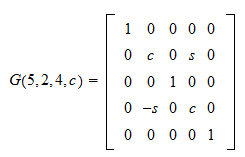In this case,,and. Thus, the matrix is obtained by modifying the second and fourth rows of aidentity matrix.

Example Another example is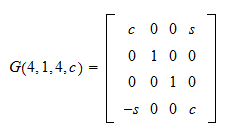which has been obtained by modifying the first and fourth rows of aidentity matrix.

Example A smaller rotation matrix follows:## Orthogonality

Givens matrices are orthogonal (i.e., their columns are orthonormal).

Proposition A Givens matrixis orthogonal, that is,Proof

LetWe need to prove thatBy using the definition of matrix product, we can see that the latter equation holds if and only ifwheredenotes the-th row of. We are going to prove that this is true. Whenand, then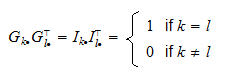because the rows of the identity matrix are orthonormal. When, thenWhen, thenWhenand(orand), so that, then we haveFinally, when eitheroris equal to one ofor(and the other one is not equal toor), we have eitherorwhich completes the proof.

## Equivalent transformations

Letbe aGivens rotation matrix.

Letbe amatrix.

What happens when we compute the productthat is, when we useto perform an equivalent transformation on?

By the usual interpretation of matrix products as linear combinations, we can see that the product is a new matrix whose rows are all equal to the corresponding rows of, except for the-th and-th. In particular, ifand, thenBut we haveExample Letand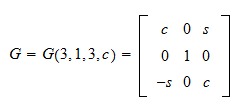Then, the product is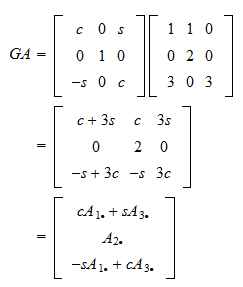## Annihilation of entries

Suppose that, as in the previous section, we perform an equivalent transformation of amatrixby using a Givens rotation.

Further suppose that.

How can we setin such a way that the transformation annihilates the entry?

We already know that, after the transformation, the-th row isTherefore,In order to have, we must haveBut we must also satisfy the constraintThese two equations are solved byExample DefineLet us find a rotation matrix that allows us to annihilate the entry. First of all, we need a non-zero entry to use as a pivot. We choose. Thus, the rows involved in the rotation are the first and the third one. As a consequence, our Givens matrix has the formThe numbersandare chosen as follows: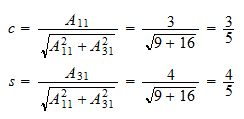Thus, the transformation is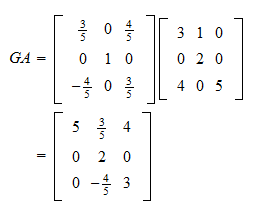which achieves the desired annihilation.

## Solved exercises

Below you can find some exercises with explained solutions.

### Exercise 1

Define the matrixFind a Givens rotation matrix that transformsinto an upper triangular matrix.

Solution

We need to annihilate the entry. We can do so by pivoting on. Thus,and. Moreover,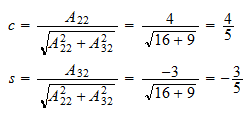Therefore, the rotation matrix isThe equivalent transformation is